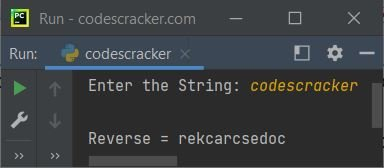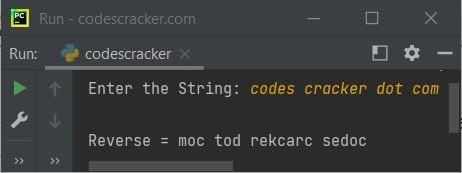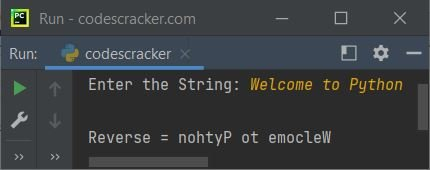# Python Program to Reverse a String

This article covers multiple programs in Python that find and prints the reverse of a string, entered by user at run-time of the program. Here are the list of programs covered in this article:

• Reverse a string in Python
• Reverse a string in Python using for loop
• Reverse a string in Python using user-defined function
• Reverse a string in Python using recursion

## Python Reverse a String

The question is, write a Python program to find and print the reverse of a given string. The program given below is its answer:

```print("Enter the String: ", end="")
str = input()

strRev = str[::-1]
str = strRev
print("\nReverse =", str)```

The snapshot given below shows the sample run of above Python program, with user input codescracker as string to find and print its reverse:Here is another sample run with user input codes cracker dot com:## Python Reverse a String using for Loop

This program does the same job as of previous program. But this program is created using for loop.

```print("Enter the String: ", end="")
str = input()

strRev = ""
for ch in str:
strRev = ch + strRev

str = strRev
print("\nReverse =", str)```

Here is its sample run with user input, Welcome to Python:## Reverse a String using Function

Here is another program in Python, that find and print the reverse of a given string, using a user-defined function named revStr():

```def revStr(s):
sReverse = s[::-1]
return sReverse

print("Enter the String: ", end="")
str = input()

str = revStr(str)
print("\nReverse =", str)```

You'll get the similar output as of previous program.

## Reverse a String using Recursion

This is the last program of this article, created using recursive function or recursion to reverse a string.

```def revStr(s):
if len(s) == 0:
return s
else:
return revStr(s[1:]) + s

print("Enter the String: ", end="")
mystr = input()

mystr = revStr(mystr)
print("\nReverse =", mystr)```

#### Same Program in Other Languages

Python Online Test

« Previous Program Next Program »